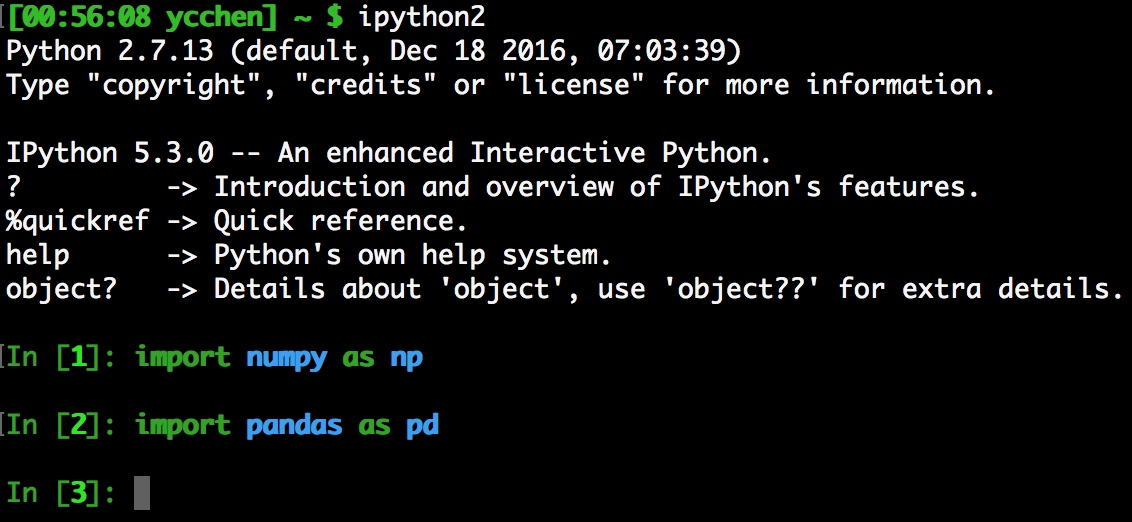# Python玩數據 (2)：Numpy [1/2]

Python常見的資料型別 / Numpy的數學運算 / Numpy基礎元素：ndarray / Numpy的矩陣運算

### IPythonIPython是一個具有互動式介面的python執行介面，你可以一邊寫一邊理解目前的狀況，舉個例子

>>> a = 12 # integer(整數)
>>> a     # check variable a
12


### Python常見的資料型別

Python常見的資料型別有整數(integer)、浮點數(floating-point number)、字串(string)、串列(list)、序對(tuple)、字典(dictionary)，可以使用type()來查詢資料型別。

>>> a = 10 # integer
>>> b = 40.0 # float, 必須有'.'
>>> c = 'word' # string
>>> d = [1, 2.0, '3'] # list
>>> e = (4, 5.0, '6') # tuple
>>> f = {'a':1, 'b':2 } # dictionary
>>> type(c)
str
>>> type(d)
list
>>> type(f)
dict


list和tuple裡面可以塞入任意的資料型別，甚至可以塞入另外一個list，或是自己定義的物件，list和tuple其實非常的相似，差異只在於tuple一旦決定了就不能在變更，但是list卻可以。

>>> d.append('new')
>>> d
[1, 2.0, '3', 'new']
>>> d = d + 1  # 取出第一項(index=0)加一再設定回去第一項
>>> d
[2, 2.0, '3', 'new']
>>> del d # 刪除index=1的那項
>>> d
[2, '3', 'new']

>>> e.append('new')   # fail
AttributeError: 'tuple' object has no attribute 'append'
>>> e
4
>>> e = e + 1   # fail
TypeError: 'tuple' object does not support item assignment
>>> del e # fail
TypeError: 'tuple' object doesn't support item deletion


>>> 1 + 2 * 2 - 6 / 2
2
>>> 1.0 + 2.0 * 2.0 - 6.0 / 2.0
2.0
>>> 3 ** 2  # 指數
9


>>> 1 / 2
0  # 整數除整數，結果必定是整是，是整數0，而不是想像中的0.5，這種運算效果有點像求商
>>> 1 / 2.
0.5 # 只要有任意浮點數出現，整數強迫轉為浮點數，然後再做運算，這才是我們要的結果
>>> 3 ** 2.
9.0


>>> 1 + '2' # fail
TypeError: unsupported operand type(s) for +: 'int' and 'str'
>>> 1 + int('2') # 使用int()將字串轉成整數
3


>>> f = (1,2,3)
>>> h = list(f)
>>> h
[1, 2, 3]
>>> h.insert(0,4) # 在index為0的地方插入整數4
>>> h
[4, 1, 2, 3]


### Numpy的數學運算

>>> np.sum([1,2,3]) # 加總
6
>>> np.max([1,2,3]) # 最大值
3
>>> np.min([1,2,3]) # 最小值
1
>>> np.mod(5,2) # 求餘數
1
>>> np.sin(np.pi/2.) # 求sin
1.0
>>> np.log(np.exp(1)) # ln 和 e
1.0


### Numpy基礎元素：ndarray

Numpy最重要的元素就是ndarray，它是N-Dimensional Array的縮寫，在Numpy裡，dimesions被稱為axes，而axes的數量被稱為rank，axes是一個重要的概念，了解這個概念基本上就把Numpy搞懂一半以上了。

>>> A = np.array([1,2,3])
>>> A
array([1, 2, 3])


>>> np.sum(A,axis=None)  # axis為None的時候則加總所有元素
6
>>> np.sum(A,axis=0)
6
>>> np.sum(A,axis=1) # fail 因為A只有一維
ValueError: 'axis' entry is out of bounds


>>> np.sum(A,axis=-1)
6


>>> B = np.array([[1,2,3],[4,5,6]])
>>> B
array([[1, 2, 3],
[4, 5, 6]])
>>> np.sum(B,axis=0)
array([5, 7, 9])   # [1+4, 2+5, 3+6]
>>> np.sum(B,axis=1)
array([ 6, 15])    # [1+2+3, 4+5+6]


>>> np.sum(B)
21
>>> np.sum(B, axis=None) # same as above
21
>>> np.sum(B, axis=-1) # 和axis=1等價
array([ 6, 15])


>>> C = np.array([[[1,2,3],[4,5,6]],[[7,8,9],[10,11,12]]])
>>> C
array([[[ 1,  2,  3],
[ 4,  5,  6]],

[[ 7,  8,  9],
[10, 11, 12]]])
>>> np.sum(C,axis=0)
array([[ 8, 10, 12],    # [1+7,  2+8,  3+9 ]
[14, 16, 18]])   # [4+10, 5+11, 6+12]
>>> np.sum(C,axis=1)
array([[ 5,  7,  9],    # [1+4, 2+5, 3+6]
[17, 19, 21]])   # [7+10, 8+11, 9+12]
>>> np.sum(C,axis=2)
array([[ 6, 15],        # [1+2+3, 4+5+6]
[24, 33]])       # [7+8+9, 10+11+12]>>> D = np.array([[1,2],[3,4],[5,6]])
>>> D
array([[1, 2],
[3, 4],
[5, 6]])
>>> D.shape
(3, 2)


(3, 2)這樣的shape我們就一點都不意外了，axis=0有三個元素，而axis=1有兩個元素。shape可以直接改，如果數量恰當的話就會自動重組。

>>> D.shape = (2,1,3)
>>> D
array([[[1, 2, 3]],

[[4, 5, 6]]])


axis=0有兩個元素，axis=1有一個元素，axis=2有三個元素。

>>> D[1, 0, 1]
5
>>> D[0, 0, 2]
3


ndarray有其資料型別，這個資料型別就稱為dtype，有哪些內建的資料型別呢？我們可以透過numpy的內建資料來查看。

>>> np.sctypes
{'complex': [numpy.complex64, numpy.complex128, numpy.complex256],
'float': [numpy.float16, numpy.float32, numpy.float64, numpy.float128],
'int': [numpy.int8, numpy.int16, numpy.int32, numpy.int64],
'others': [bool, object, str, unicode, numpy.void],
'uint': [numpy.uint8, numpy.uint16, numpy.uint32, numpy.uint64]}


>>> t1 = np.array([1,2,3],dtype='int32')
>>> t1
array([1, 2, 3], dtype=int32)
>>> t1.dtype
dtype('int32')
>>> t2 = np.array([1,2,3],dtype='float64')
>>> t2
array([ 1.,  2.,  3.])
>>> t2.dtype
dtype('float64')


### Numpy的矩陣運算

>>> A = np.array([[1,2],[3,4]],dtype='float64')
>>> B = np.array([[5,0],[0,0]],dtype='float64')
>>> A+B      # element-wise plus
array([[ 6.,  2.],
[ 3.,  4.]])


A和B矩陣中同樣位置的元素相加，再放到新的矩陣中，這一種操作就叫做element-wise operation。

>>> A-B      # element-wise minus
array([[-4.,  2.],
[ 3.,  4.]])
>>> A*B      # element-wise multiply
array([[ 5.,  0.],
[ 0.,  0.]])
>>> B/A      # element-wise divide
array([[ 5.,  0.],
[ 0.,  0.]])


>>> np.dot(A,B) # 矩陣內積
array([[  5.,   0.],
[ 15.,   0.]])


>>> A = np.array([[1,2],[3,4]],dtype='float64')
>>> A
array([[ 1.,  2.],
[ 3.,  4.]])
>>> A.T # 矩陣轉置
array([[ 1.,  3.],
[ 2.,  4.]])


>>> A_rev = np.linalg.inv(A) # 反矩陣
>>> A_rev
array([[-2. ,  1. ],
[ 1.5, -0.5]])
>>> np.dot(A,A_rev)
array([[  1.00000000e+00,   0.00000000e+00],
[  8.88178420e-16,   1.00000000e+00]])


A和A的反矩陣內積為單位矩陣，你有注意到8.88178420e-16這個奇怪的數字嗎？這是因為python在計算的過程有一些誤差的緣故，所以才會產生一個這麼小的數字，但基本上可以看作是0。

>>> A = np.array([[1,2],[3,4]],dtype='float64')
>>> B = np.array([[5,0],[0,0]],dtype='float64')
>>> V = np.vstack((A,B))
>>> V
array([[ 1.,  2.],
[ 3.,  4.],
[ 5.,  0.],
[ 0.,  0.]])


>>> H = np.hstack((A,B))
>>> H
array([[ 1.,  2.,  5.,  0.],
[ 3.,  4.,  0.,  0.]])


>>> np.vsplit(V,2)  # 2代表切兩份
[array([[ 1.,  2.],
[ 3.,  4.]]), array([[ 5.,  0.],
[ 0.,  0.]])]


>>> np.hsplit(H,4)  # 4代表切四份
[array([[ 1.],
[ 3.]]), array([[ 2.],
[ 4.]]), array([[ 5.],
[ 0.]]), array([[ 0.],
[ 0.]])]


### 子彈總結

• Python常見的資料型別：整數(integer)、浮點數(floating-point number)、字串(string)、串列(list)、序對(tuple)、字典(dictionary)
• ndarray的axes概念很重要，這會決定函數操作的方式，例如：np.sum
• ndarray的資料型別(dtype)，例如：'float64', 'int64', 'string', ...
• numpy的矩陣運算有element-wise operation和matrix operation兩種